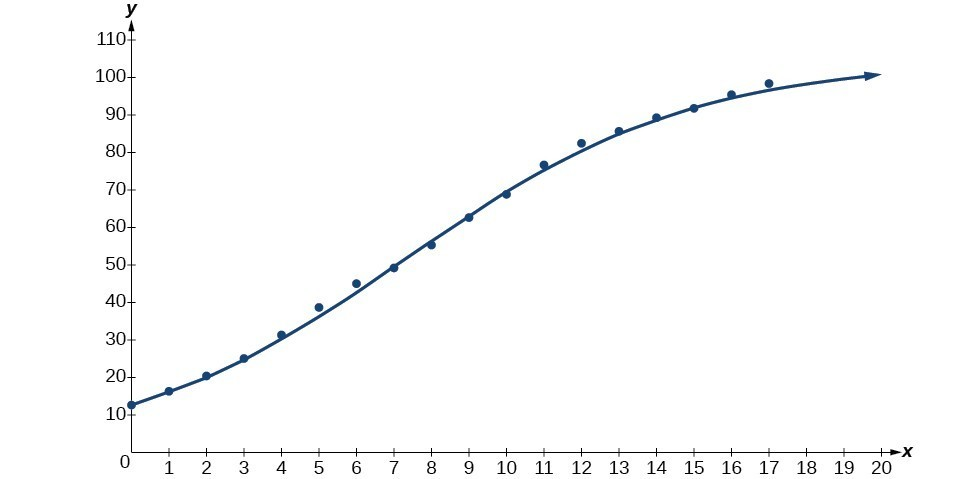## Build a logistic model from data

Like exponential and logarithmic growth, logistic growth increases over time. One of the most notable differences with logistic growth models is that, at a certain point, growth steadily slows and the function approaches an upper bound, or limiting value. Because of this, logistic regression is best for modeling phenomena where there are limits in expansion, such as availability of living space or nutrients.

It is worth pointing out that logistic functions actually model resource-limited exponential growth. There are many examples of this type of growth in real-world situations, including population growth and spread of disease, rumors, and even stains in fabric. When performing logistic regression analysis, we use the form most commonly used on graphing utilities:

$y=\frac{c}{1+a{e}^{-bx}}$

Recall that:

• $\frac{c}{1+a}$ is the initial value of the model.
• when > 0, the model increases rapidly at first until it reaches its point of maximum growth rate, $\left(\frac{\mathrm{ln}\left(a\right)}{b},\frac{c}{2}\right)$. At that point, growth steadily slows and the function becomes asymptotic to the upper bound = c.
• c is the limiting value, sometimes called the carrying capacity, of the model.

### A General Note: Logistic Regression

Logistic regression is used to model situations where growth accelerates rapidly at first and then steadily slows to an upper limit. We use the command “Logistic” on a graphing utility to fit a logistic function to a set of data points. This returns an equation of the form

$y=\frac{c}{1+a{e}^{-bx}}$

Note that

• The initial value of the model is $\frac{c}{1+a}$.
• Output values for the model grow closer and closer to y = c as time increases.

### How To: Given a set of data, perform logistic regression using a graphing utility.

1. Use the STAT then EDIT menu to enter given data.
1. Clear any existing data from the lists.
2. List the input values in the L1 column.
3. List the output values in the L2 column.
2. Graph and observe a scatter plot of the data using the STATPLOT feature.
1. Use ZOOM  to adjust axes to fit the data.
2. Verify the data follow a logistic pattern.
3. Find the equation that models the data.
1. Select “Logistic” from the STAT then CALC menu.
2. Use the values returned for a, b, and c to record the model, $y=\frac{c}{1+a{e}^{-bx}}$.
4. Graph the model in the same window as the scatterplot to verify it is a good fit for the data.

### Example 3: Using Logistic Regression to Fit a Model to Data

Mobile telephone service has increased rapidly in America since the mid 1990s. Today, almost all residents have cellular service. The table below shows the percentage of Americans with cellular service between the years 1995 and 2012.

Year Americans with Cellular Service (%) Year Americans with Cellular Service (%)
1995 12.69 2004 62.852
1996 16.35 2005 68.63
1997 20.29 2006 76.64
1998 25.08 2007 82.47
1999 30.81 2008 85.68
2000 38.75 2009 89.14
2001 45.00 2010 91.86
2002 49.16 2011 95.28
2003 55.15 2012 98.17
1. Let x represent time in years starting with = 0 for the year 1995. Let y represent the corresponding percentage of residents with cellular service. Use logistic regression to fit a model to these data.
2. Use the model to calculate the percentage of Americans with cell service in the year 2013. Round to the nearest tenth of a percent.
3. Discuss the value returned for the upper limit, c. What does this tell you about the model? What would the limiting value be if the model were exact?

### Solution

1. Using the STAT then EDIT menu on a graphing utility, list the years using values 0–15 in L1 and the corresponding percentage in L2. Then use the STATPLOT feature to verify that the scatterplot follows a logistic pattern as shown in Figure 5:

Use the “Logistic” command from the STAT then CALC menu to obtain the logistic model,

$y=\frac{105.7379526}{1+6.88328979{e}^{-0.2595440013x}}$Figure 6

Next, graph the model in the same window as shown in Figure 6 to verify it is a good fit:

2. To approximate the percentage of Americans with cellular service in the year 2013, substitute = 18 for the in the model and solve for y:

$\begin{cases}y\hfill & =\frac{105.7379526}{1+6.88328979{e}^{-0.2595440013x}}\hfill & \text{Use the regression model found in part (a)}.\hfill \\ \hfill & =\frac{105.7379526}{1+6.88328979{e}^{-0.2595440013\left(18\right)}}\hfill & \text{Substitute 18 for }x.\hfill \\ \hfill & \approx \text{99}\text{.3 }\hfill & \text{Round to the nearest tenth}\hfill \end{cases}$

According to the model, about 98.8% of Americans had cellular service in 2013.

3. The model gives a limiting value of about 105. This means that the maximum possible percentage of Americans with cellular service would be 105%, which is impossible. (How could over 100% of a population have cellular service?) If the model were exact, the limiting value would be = 100 and the model’s outputs would get very close to, but never actually reach 100%. After all, there will always be someone out there without cellular service!

### Try It 3

The table below shows the population, in thousands, of harbor seals in the Wadden Sea over the years 1997 to 2012.

Year Seal Population (Thousands) Year Seal Population (Thousands)
1997 3.493 2005 19.590
1998 5.282 2006 21.955
1999 6.357 2007 22.862
2000 9.201 2008 23.869
2001 11.224 2009 24.243
2002 12.964 2010 24.344
2003 16.226 2011 24.919
2004 18.137 2012 25.108

a. Let x represent time in years starting with x = 0 for the year 1997. Let y represent the number of seals in thousands. Use logistic regression to fit a model to these data.

b. Use the model to predict the seal population for the year 2020.

c. To the nearest whole number, what is the limiting value of this model?

Solution

1. Source: The World Bank, 2013